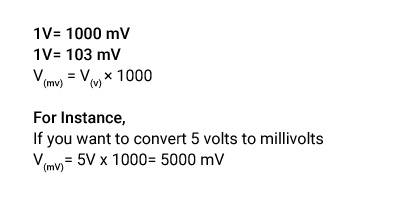## About Our V to mV Converter

"Learning Never Exhausts The Mind, But A Boring Way Of Learning Can Definitely Exhaust The Mind."

You can now access a handy conversion calculator for quick calculations regardless of the size of numbers and units. We proudly present a converter that will not only save you time but will also provide accurate outcomes. Whether you want to convert volts to millivolts or other metric conversions, get the results straight away.

### How to Convert Volts to Millivolts?

You must multiply the electric potential value by the conversion ratio to convert volts to millivolts. One Volt is equal to 1000 millivolts.

### The Volts to Millivolts Conversion Formula.

Do a quick volt to millivolts conversion using a straight formula.The most comforting prospect is you can do any metric conversion with the help of our converter. You can view more details on each measurement unit on our page.

### How to Use Our Volts to Millivolts Converter?

You can change Volts to millivolts on the count of three.

• Step1: Land on our website.
• Step2: Enter the voltage value that you want to convert to millivolts.
• Step3: Press the "Convert" button, and you will get the answer straight away.

Our Volt to millivolt calculator will save time and give you a quick outcome.

### How Many Volts Are in a Millivolt?

1 Millivolt = 1/1000 Volts

1 mV = 103 mV = 0.001 V

One millivolt is equal to a thousandth part of a volt.

### What is the Difference Between Volts & Millivolts?

A volt is a SI unit that measures the electrical potential difference and electromotive force. One Volt is equal to 1,000 millivolts. Volt is represented by V.

A millivolt is also an SI-derived unit for Voltage, but a millivolt is used when there is a requirement for smaller units. A millivolt is the 1/1,000 part of a volt and is denoted by mV.

### Examples of Converting Volts to Millivolts.

Have a look at some examples to help you convert the volts to millivolts.

V(mV) = 1 V x 103 mV= 1,000 mV

V(mV) = 6 V x 103 mV= 6,000 mV

V(mV) = 9V x 103 mV= 9,000 mV

### Volt to Millivolts Conversion Table.

Have a quick look at the Volt to millivolt conversion table,

Volts Millivolts
0.001V 1mV
0.01V 10mV
0.1V 100mV
1V 1000mV
10V 10000mV
100V 100000mV